# Solutions to SQA examination

## 2005

```Section A
1. A		11. E
2. E		12. C
3. C		13. B
4. B		14. B
5. E		15. A
6. B		16. A
7. C		17. D
8. D		18. D
9. E		19. B
10.C		20. D

Section B

21.a.i.	(A) Mean = sum/N
Mean = (0.015 + 0.013 + 0.014 + 0.019 + 0.017 + 0.018)/6
Mean =  0.096/6
Mean =  0.016s

(B) RND Uncertainty = (Max - Min)/N
RND Uncertainty = (0.019 - 0.013)/6
RND Uncertainty = 0.006/6
RND Uncertainty = 0.001s

a.ii.	u = 0m/s
v = 1.25m/s	[ v = card width/t = 0.020/0.016 = 1.25m/s]
s = 0.60m

Use: 	v2 = u2 +2as
a = (v2 -u2)/2s
a = (1.252 - 0)/2x0.6
a = 1.5625/1.2
a = 1.30m/s2

b.i.	Photoconducting mode.

ii.	When the beam is broken the photodiode is in
darkness and does not conduct. This increases
the voltage at point Y. When this voltage reaches
2.0V or higher the MOSFET conducts and starts the
timer.

22.a.i.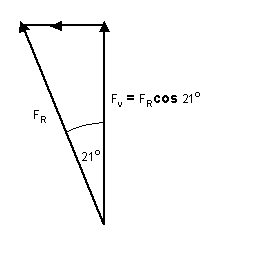FV(total) = 2FRcos21o
FV(total) = 2 x 4.5x103cos21o
FV(total) = 8402.22N

a.ii.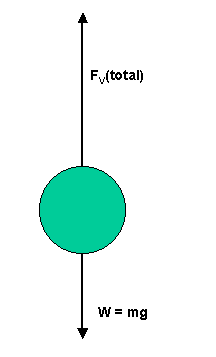Fun = FV(total) - mg
Fun = 8402.22 - (236 x 9.8)
Fun = 6089.42N

a = Fun/m
a = 6089.42/236
a = 25.8m/s2

b.	The tension in the cords decrease as the
capsule rises. This means the total upward
force and therefore the unbalanced force
decreases, reducing the acceleration.

c.	When there is no upward tension force the
seat and occupants will both be projectiles
in free fall. Both will be accelerating
downwards at a rate of 9.8m/s2.

23.a.	m = 12g = 12x10-3kg
v = (0.5x0.3x0.1)m3 = 0.015m3

r = m/v
r = 12x10-3kg/0.015m3
r = 0.8kg/m3

b.	Pressure is directly proportional to the depth.
This means that the lower surface has a greater
pressure acting on it than the upper surface.
The resulting forces (F = PA) are shown in the
diagram.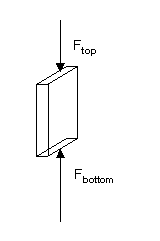Fbottom is greater than Ftop.

Fupthrust = Fbottom - Ftop

c.	The initial accelerating force is given by:
Fun = Fupthrust - weight(w)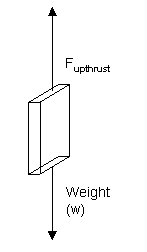However, as the float accelerates upwards and its
speed increases the water resistance force also
increases. This means the acceleration of the float
decreases.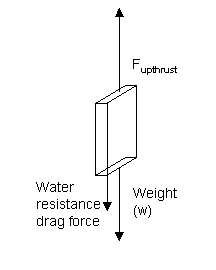Fun = Fupthrust - (weight(w) + water resistance)

d.	As the density is greater the mass and weight
of the float is greater. The upward unbalanced force:

Fun = Fupthrust - weight(w)  ...is less.

The acceleration :

a = Fun/m   ...is also less.

24.a.i.

Temperature/oC
25
50
75
100

Temperature/k
298
323
348
373

Volume/ml
20.6
22.6
24.0
25.4

(Volume/Temperature)/(ml/k)
0.0691
0.0700
0.0690
0.0681

Volume/Temperature(k) = constant
Volume = constant x Temperature(k)
Volume varies directly with the kelvin temperature.

a.ii.	T(k) = (65 +273)k = 338k
Constant = 0.69ml/k

Volume = constant x Temperature(k)
Volume = 0.069 x 338
Volume = 23.3ml

a.iii.	As the temperature of the gas increases the kinetic
energy and speed of the gas particles increase. This
means that the average force, per collision, of the
gas particles with the container walls increases. This
produces an upward force on the syringe causing it to
move upward until the pressure inside is the same as
the air pressure outside.

b.i.	This is a wheatstone bridge circuit and is balanced when

General equation: R1/R2 = R3/R4

Rvariable/500 = 2000/1000
Rvariable = 500 x 2
Rvariable = 1000W

b.ii.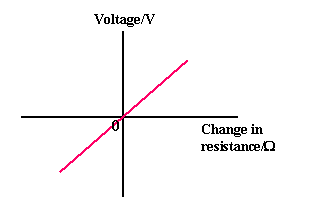25.a.	e.m.f
The open circuit voltage.
OR
The energy supplied by the battery to each coulomb of charge.

b.i.	(A) The e.m.f. is found at the y-intercept.
e.m.f. = 6.0V.

(B) The internal resistance(r) is equal to the negative gradient (-m)

m = (y2 - y1)/(x2 - x1)
m =  (6.0 - 1.0)/(0 - 1.0)
m = -5

r = -m = -(-5) = 5W

b.ii.	E = I(R + r)
R = (E/I) - r
R = (6/0.3) - 5
R = 20 - 5 = 15W ...as required.

c.	1/Rp = 1/R1 + 1/R2
1/Rp = 1/15 + 1/30
1/Rp = 3/30
Rp = 10W

I = E/(Rp + r)
I = 6.0/(10 + 5)
I = 6.0/15
I = 0.4A

26.a.i.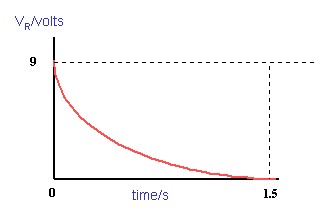a.ii.	The charging time is longer because less charge is
transferred per second with a higher value resistor.

a.iii.	Q = CVC

Vsupply = VC + VR
VC = Vsupply - VR
VC = 9 - 4
VC = 5V

Q = 2200x10-6 x 5
Q = 11000x10-6C  (11mC)

b.i.	Maximum energy is stored when: VC = Vsupply = 9V

Emax = 1/2(CVC2)
Emax = 1/2(2200x10-6 x 92)
Emax = 1100x10-6 x 81
Emax = 89100x10-6J  (89.1mJ)

b.ii.	The maximum discharge current occurs when the
maximum voltage across the capacitor is applied
across the resistor.

Imax = V(R)max/R
Imax = 9/100x103
Imax = 0.00009A  (90mA)

27.a.	x = source
y = gate
z = drain

b.i.	Vout = Rf/R1(V2 - V1)
2 = 1x106/500x103(V2 - V1)
(V2 - V1) = 2/2
(V2 - V1) = 1V

b.ii.	V1 = VQ
V2 = VP

V2 = V1 + 1
VP = VQ + 1

The voltage at Q can be solved by proportion.

VQ =[R20kW/(R20kW + R100kW)]Vsupply
VQ =[20kW/(20kW + 100kW)]Vsupply
VQ =[20kW/120kW]Vsupply
VQ =[(1/6)]12
VQ = 2V

VP = VQ + 1
VP = 2 + 1
VP = 3V (voltage across thermistor)

VP/V75kW = Rthermistor/R75kW
3/V75kW = Rthermistor/75kW

V75kW = 9V  [as Vsupply = VP + V75kW]
3/9 = Rthermistor/75kW

Rthermistor = 1/3(75kW)
Rthermistor = 25kW

28.a.i.	n =singlass = sinqair/sinqglass
nglass = sin47o/sin29o
nglass = 1.51

a.ii.	sinqair = nglasssinglass
sinqair = 1.51xsin31o
sinqair = 0.778
qair = 51.1o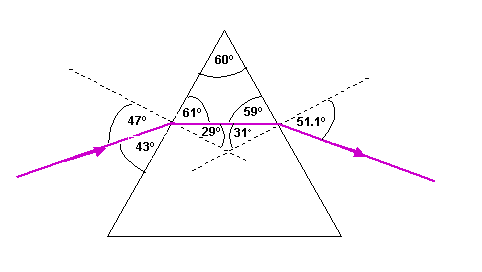b.i.	When the path difference between adjacent slits to a
point on the screen is zero or a whole number of
wavelengths a bright spot is produced because of
constructive interference.

b.ii.	dsinq = nl

300 lines/mm => 300000 lines/m
d = 1/300000 = 3.3333x10-6m
n = 2 (second order)

sinq = nl/d
sinq = 2 x 650x10-9/3.3333x10-6
sinq = 0.390
q  = 22.95o

b.iii.	The blue fringes are closer together because shorter wavelength
blue light is diffracted less than the longer wavelength
red light.

29.a.	Above the threshold frequency the photoelectric current
is directly proportional to the intensity of radiation.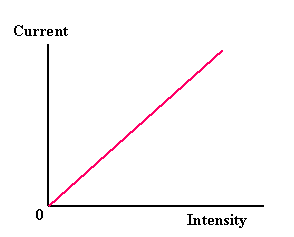b.i.	Ek(max) = Ephoton - Ework function

fphoton = v/l
fphoton = 3x108/400x10-9
fphoton = 7.5x1014Hz

Ephoton = hf
Ephoton = 6.63x10-34 x 7.5x1014
Ephoton = 4.9725-19J

Ek(max) = 4.9725x10-19 - 3.11x10-19
Ek(max) = 1.8625x10-19J

b.ii.	The photons may have a kinetic energy greater than the
work the electric field does trying to stop them.

Ek(max) > eV	  [V = potential difference applied by battery
e = electronic charge]

30.a.i.	At 200cpm material thickness = 1mm
At 100cpm material thickness = 6mm

5mm of material is required to half the count.
This is the half value thickness.

a.ii.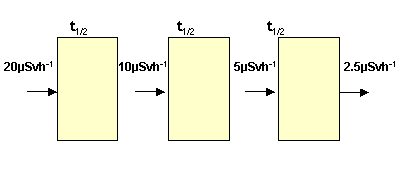Three half value thicknesses are required.
Thickness = 3 x 5 = 15mm

b.	Htotal/h = Hg/h + Hthermal neutrons/h + Hfast neutrons/h

Hg/h = QgDg/h
Hg/h = 1 x 2.0mGy/h
Hg/h = 2.0mSv/h

Hthermal neutrons/h = Qthermal neutronsDthermal neutrons/h
Hthermal neutrons/h = 3 x 400mGy/h
Hthermal neutrons/h = 1200mSv/h = 1.2mSv/h

Hfast neutrons/h = Qfast neutronsDfast neutrons/h
Hfast neutrons/h = 10 x 80mGy/h
Hfast neutrons/h = 800mSv/h = 0.8mSv/h

Htotal/h = (2.0 + 1.2 + 0.8)mSv/h
Htotal/h = 4.0mSv/h

thours = 500/4
thours = 125hours

END OF QUESTION PAPER